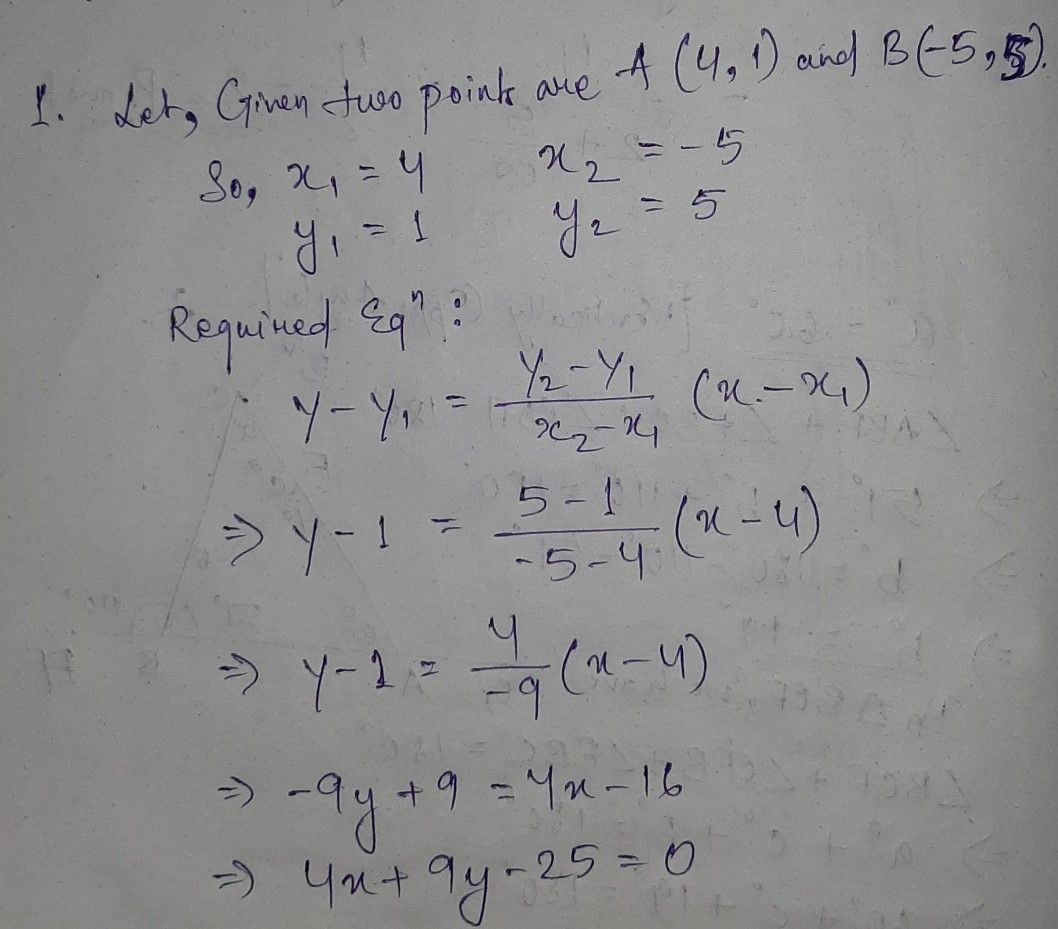Symbol
ProblemLearning Task $4:$ Do the following in your notebook. A. Find the equation of the line given the graph. $1$ $2$ $3$